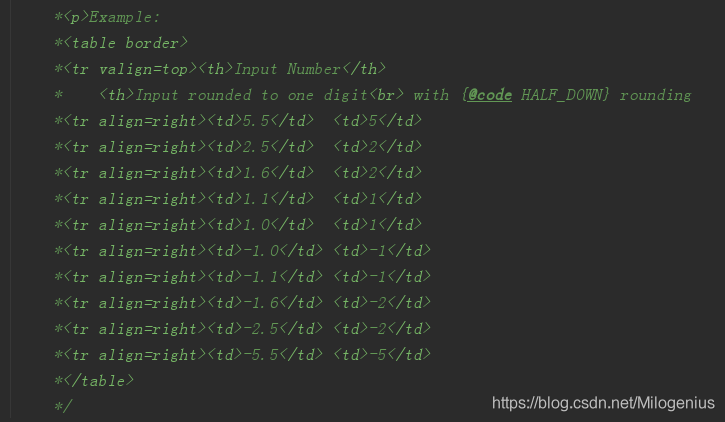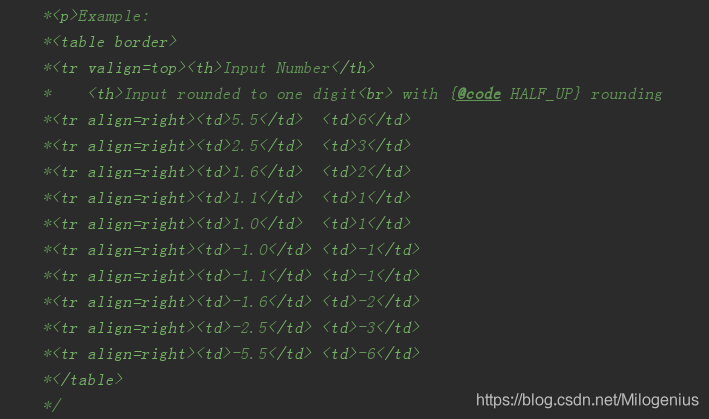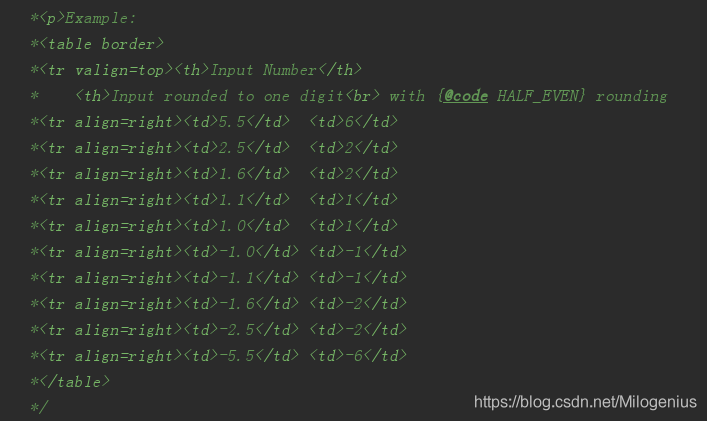Java中的浮点数四舍五入到小数点后2位的几种方法麦洛466 0 0

## 相关知识点

### 1.Java中的舍入模式

RoundingMode.HALF_DOWN：如果两个邻居等距，则向下舍入，例如2.5将舍入为2.0RoundingMode.HALF_UP：如果两个邻居的距离相同则向上舍入，例如2.5将舍入为3.0RoundingMode.HALF_EVEN：如果两个邻居等距，则向偶数舍入，例如2.5将舍入到2.0，而5.5将舍入到6.0### 2.使用BigDecimal将数字四舍五入到小数点后两位

package com.milo.base.sort;

import org.junit.Test;

import java.math.BigDecimal;

/**
* 测试BigDecimal的Api
* @author milogenius
* @date 2020-04-14 19:49
*/
public class BigDecimalTest {

@Test
public void test1(){
float number = BigDecimal.valueOf(4.526f)
.setScale(2, BigDecimal.ROUND_HALF_DOWN)
.floatValue();
System.out.println(number);
}
}
----------------------------------------------
Output:
4.53

setScale（）中，您可以指定需要舍入的小数位数，在这里，我们将小数位数设置为2，因为我们将舍入到2位数字。 我们指定舍入模式ROUND_HALF_DOWN，类似于RoundingMode.HALF_DOWN。 您也可以使用RoundingMode.HALF_DOWN来代替BigDecimal.ROUND_HALF_DOWN，但是要注意，从Java 5开始添加了RoundingMode枚举。

### 3.使用DecimalFormat将数字四舍五入到小数点后两位

    @Test
public void test2(){
DecimalFormat df = new DecimalFormat("#.00");
float number = Float.valueOf(df.format(4.526f));
System.out.println(number);
}

----------------------------------------------
Output:
4.53


＃.00用于四舍五入到小数点后两位，如果要四舍五入到小数点后第三位，只需使用＃.000＃.0000来创建DecimalFormat。 要了解有关格式化数字的更多信息，请参见如何使用DecimalFormat在Java中格式化数字。

### 4.使用Math.round（）在Java中进行舍入运算

Math.round（）是Java中舍入数字的经典方法。 尽管它没有提供任何API来指定要舍入的位数，但是通过使用乘法和除法，您可以将其舍入到n或2个小数位。

    @Test
public void test3(){
float rounded = (float) Math.round(4.526f*100)/100;
System.out.println(rounded);
}
----------------------------------------------
Output:
4.53

## 综合案例

package com.milo.base.sort;

import java.math.BigDecimal;
import java.text.DecimalFormat;
import java.util.Arrays;

/**
* 使用三种方式演示java中保留2位小数
* 包括 BigDecimal,
* Math.round and DecimalFormat class.
* @author milogenius
*/

public class RoundingNumbers {

public static void main(String args[]) {

//数据
float[] samples = new float[]{2.123f, 2.125f, 2.127f};

//使用 Match.round()保留2位小数
float[] rounded = round(samples);

System.out.println("开始舍入, numbers: "
+ Arrays.toString(samples));
System.out.println("结束舍入 : 使用Match.round()方法 "
+ Arrays.toString(rounded));

//使用BigDecimal保留2位小数
//我们可以使用不同的舍入模式e.g., HALF_UP, HALF_DOWN,and HALF_EVEN with BigDecimal
rounded = roundUsingBigDecimal(samples);

System.out.println("开始舍入,numbers : "
+ Arrays.toString(samples));

System.out.println("结束舍入, number 使用 BigDecimal: "
+ Arrays.toString(rounded));

//DecimalFormat Example to round number to 2 digits
//使用DecimalFormat来保留2位小数
rounded = roundUsingDecimalFormat(samples);
System.out.println("开始舍入numbers: "
+ Arrays.toString(samples));
System.out.println("结束舍入,使用DecimalFormat: "
+ Arrays.toString(rounded));

}

/**
*  使用 Math.round保留2为小数
* @param numbers
* @return
*/
public static float[] round(float[] numbers) {

float[] round = new float[numbers.length];
for (int i = 0; i < numbers.length; i++) {
float number = numbers[i];
round[i] = (float) Math.round(number * 100) / 100;
}
return round;
}

/**
* 使用BigDecimal保留2为小数
* @param digits
* @return
*/
public static float[] roundUsingBigDecimal(float[] digits) {
float[] result = new float[digits.length];
for (int i = 0; i < digits.length; i++) {
float digit = digits[i];
result[i] = BigDecimal.valueOf(digit)
.setScale(2, BigDecimal.ROUND_HALF_DOWN)
.floatValue();
}
return result;
}

/**
* 使用DecimalFormat保留2为小数
* @param decimals
* @return
*/
public static float[] roundUsingDecimalFormat(float[] decimals) {
float[] rounded = new float[decimals.length];
//DecimalFormat to round numbers to 2 decimal place
DecimalFormat df = new DecimalFormat("#.00");
for (int i = 0; i < decimals.length; i++) {
float decimal = decimals[i];
rounded[i] = Float.valueOf(df.format(decimal));
}
return rounded;
}
}